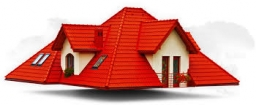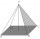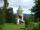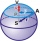# The roof

The roof of the tower has the shape of a regular quadrangular pyramid, the base edge of which is 11 m long and the side wall of the animal with the base an angle of 57°. Calculate how much roofing we need to cover the entire roof, if we count on 15% waste.

x =  255.4903 m2

### Step-by-step explanation:Did you find an error or inaccuracy? Feel free to write us. Thank you!Tips to related online calculators

#### You need to know the following knowledge to solve this word math problem:

We encourage you to watch this tutorial video on this math problem:

## Related math problems and questions:

• House roofThe roof of the house has the shape of a regular quadrangular pyramid with a base edge 17 m. How many m2 is needed to cover roof if roof pitch is 57° and we calculate 11% of waste, connections and overlapping of area roof?How many square meters are needed to cover the shape of a regular quadrangular pyramid base edge 10 meters if the deviation lateral edges from the base plane are 68°? Calculate waste 10%.
• TowerThe top of the tower is a regular hexagonal pyramid with base edge 8 meters long and a height 5 meters. How many m2 of the sheet is required to cover the top of the tower if we count 8% of the sheet waste?
• Pyramid 8Calculate the volume and the surface area of a regular quadrangular pyramid with the base side 9 cm and side wall with the base has an angle 75°.
• Base diagonalIn a regular 4-sided pyramid, the side edge forms an angle of 55° with the base's diagonal. The length of the side edge is eight meters. Calculate the surface area and volume of the pyramid.
• The bus stopThe bus stop waiting room has the shape of a regular quadrilateral pyramid 4 m high with a 5 m base edge. Calculate how much m2 roofing is required to cover the sheathing three walls, taking 40% of the additional coverage.
• Roof coverAbove the pavilion with a square ground plan with a side length of a = 12 m is a pyramid-shaped roof with a height v = 4.5 m. Calculate how much m2 of sheet metal is needed to cover this roof if 5.5% of the sheet we must add for joints and waste.
• Roof 7The roof has the shape of a regular quadrangular pyramid with a base edge of 12 m and a height of 4 m. How many percent is folds and waste if in construction was consumed 181.4m2 of plate?
• ConeCalculate volume and surface area of ​​the cone with a diameter of the base d=15 cm and side of the cone with the base has angle 52°.
• Roof 8How many liters of air are under the roof of the tower, which has the shape of a regular six-sided pyramid with a 3,6-meter-long bottom edge and a 2,5-meter height? Calculate the supporting columns occupy about 7% of the volume under the roof.
• Pyramid roof2/4 of the area of ​​the roof-shaped regular tetrahedral pyramid with base edge 10 m and height of 4 m is already covered with roofing. How many square meters still need to be covered?
• Top of the towerThe top of the tower has the shape of a regular hexagonal pyramid. The base edge has a length of 1.2 m, the pyramid height is 1.6 m. How many square meters of sheet metal is needed to cover the top of the tower if 15% extra sheet metal is needed for joint
• The tentThe tent shape of a regular quadrilateral pyramid has a base edge length a = 2 m and a height v = 1.8 m. How many m2 of cloth we need to make the tent if we have to add 7% of the seams? How many m3 of air will be in the tent?
• The roofThe roof has a spherical canopy with a base diameter of 8 m and a height of 2 m. Calculate the foil area with which the roof is covered when calculating 13% for waste and residues.
• Pyramid - angleCalculate the regular quadrangular pyramid's surface whose base edge measured 6 cm, and the deviation from the plane of the base's sidewall plane is 50 degrees.
• Church roof 2The roof has the shape of a rotating cone shell with a base diameter of 6 m and a height of 2.5 m. How many monez (CZK) will cost the roof cover sheet if 1 m2 of metal sheet costs 152 CZK and if you need 15% extra for joints, overlays and waste?
• Hexagonal pyramidCalculate the volume and surface area of a regular hexagonal pyramid with a base edge a = 30 m and a side edge b = 50 m.